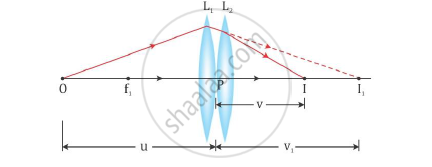Share

# Two Thin Convex Lenses L1 and L2 of Focal Lengths F1 and F2, Respectively, Are Placed Coaxially in Contact. an Object is Placed at a Point Beyond the Focus of Lens L1. - CBSE (Science) Class 12 - Physics

ConceptRefraction at Spherical Surfaces and by Lenses Combination of Thin Lenses in Contact

#### Questions

Two thin convex lenses L1 and L2 of focal lengths f1 and f2, respectively, are placed coaxially in contact. An object is placed at a point beyond the focus of lens L1. Draw a ray diagram to show the image formation by the combination and hence derive the expression for the focal length of the combined system.

Draw a ray diagram to show the image formation by a combination of two thin convex lenses in contact. Obtain the expression for the power of this combination in terms of the focal lengths of the lenses.

#### Solution

Consider two thin lens L1 and L2 of focal length f1 and f2 held coaxially in contact with each other. Let P be the point where the optical centres of the lenses coincide (lenses being thin).

Let the object be placed at a point O beyond the focus of lens L1 such that OP = u (object distance). Lens L1 alone forms the image at I1 where P I1 = v1 (image distance). The image I1 would serve as a virtual object for lens L2 which forms a final image I at distance PI = v. The ray diagram showing the image formation by the combination of these two thin convex lenses will be as shown below:From the lens formula, for the image I1 formed by the lens L1, we have

1/v_1-1/u=1/f_1

For the image formation by the second lens, L2

1/v-1/v_1=1/f_2 """...........2"

Adding (1) and (2) we get:

1/v_1-1/u+1/v-1/v_1=1/f_1+1/f_2

-1/u+1/v=1/f_1+1/f_2

1/v-1/u=1/f_1+1/f_2

If the two lenses are considered a single lens of focal length f, which forms an image I at a distance v with an object distance being u, then we get

1/v-1/u=1/f """ (where"1/f=1/f_1+1/f_2)

=>f=(f_1f_2)/(f_1+f_2)

Hence, the focal length of the combined system is given by f=(f_1f_2)/(f_1+f_2)

In terms of power, equation can be written as

P = 1/f_1 + 1/f_2   (as P = 1/f)

Is there an error in this question or solution?

#### APPEARS IN

Solution Two Thin Convex Lenses L1 and L2 of Focal Lengths F1 and F2, Respectively, Are Placed Coaxially in Contact. an Object is Placed at a Point Beyond the Focus of Lens L1. Concept: Refraction at Spherical Surfaces and by Lenses - Combination of Thin Lenses in Contact.
S